# Java基础第九讲：面向对象基础（三）

#### Java基础第九讲：面向对象基础（三）

 `1` `public` `class` `Lesson09 {`
 `2`
 `3` `}`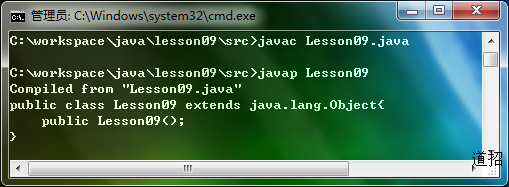5、继承的原则：
1. 子类能够继承父类中被声明为public和protected的成员变量和成员方法。
2. 子类能够继承在同一个包中的默认修饰符修饰的成员变量和成员方法。
3. 如果子类声明了一个与父类变量同名的成员变量，则子类不能继承父类的成员变量，这种做法叫做变量的隐藏。
4. 如果子类声明了一个与父类方法同名的成员方法，则子类不能继承父类的成员方法，这种做法方法的重写。
二、包 package 1、编译单元(compilation unit) 在Java里，一个编译单元就是一个用来书写Java源代码的文本文件。我们前面讲类的定义的时候只关注了类内部的东西，类外面是不是也有东西？答案是肯定的。编译单元有三个组成部分：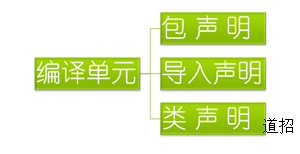这三个部分都是可选的，包声明如果要有必须写在最前面，并且只能写一份。导入声明可以写多个，类声明也可以写多个。 2、包的概念(package) 类名是类之间彼此区分的标示，一个程序中类数量增多是，必然会遇到类名冲突的情形。包提供了类的组织和管理方式。包的用途有以下三种：
1. 将功能相近的类放在同一个包里，方便使用和查找
2. 类名相同的文件可以放在不同的包里而不会产生冲突
3. 可以依据包设定访问权限
3、包的声明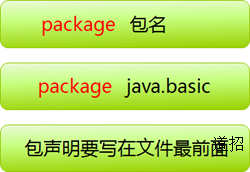4、 包的例子：
 `01` `//包的声明`
 `02` `package` `android.java.basic;`
 `03`
 `04` `//导入声明`
 `05` `import` `java.util.Date;`
 `06`
 `07` `//类声明`
 `08` `class` `Animal{`
 `09` `    ``long` `birthTime = ``new` `Date().getTime();`
 `10`
 `11` `    ``void` `eat(){`
 `12` `        ``System.out.println(``"eating"``);`
 `13` `    ``}`
 `14` `}`
 `15`
 `16` `//类声明`
 `17` `class` `Fish ``extends` `Animal {`
 `18` `    ``void` `swim(){`
 `19` `        ``System.out.println(``"swimming"``);`
 `20` `    ``}`
 `21` `}`
 `22`
 `23` `//类声明`
 `24` `public` `class` `Lesson09 {`
 `25` `    ``public` `static` `void` `main(String[] args){`
 `26`
 `27` `        ``//动物类`
 `28` `        ``Animal a = ``new` `Animal();`
 `29` `        ``a.eat();`
 `30` `        ``System.out.println(a.birthTime);`
 `31`
 `32` `        ``//鱼类`
 `33` `        ``Fish f = ``new` `Fish();`
 `34` `        ``f.eat();`
 `35` `        ``f.swim();`
 `36` `        ``System.out.println(f.birthTime);`
 `37` `    ``}`
 `38` `}`

 看见性 public protected 默认 private 从同一个类 是 是 是 是 从同一个包中的任何类 是 是 是 否 从同一个包中的子类 是 是 是 否 从包外的子类 是 是，通过继承 否 否 从包外的任何非子类的类 是 否 否 否
1. 对于类的修饰符，只能有2个选择，用public修饰或者不用（不用就是默认修饰符）。
2. 如果一个类本身对另一个类不可见，则即使将其成员声明为public，也没有一个成员是可见的，只有当你确类本身对你是可见的时，查看其各个成员的访问级别才有意义。
3. 对于类的成员（member, 包括属性和方法），可以用 public protected 默认的和private 4种修饰符。
4. 永远不要用访问修饰符修饰局部变量，编译器会毫不留情的报错。（记住：局部变量只有一个修饰符可以用，那就是final）

 `01` `public` `class` `Lesson09_1 {`
 `02`
 `03` `    ``int` `i=``1``;`
 `04` `    ``int` `j=``1``;`
 `05` `    ``int` `k=``1``;`
 `06`
 `07` `    ``void` `test(``int` `i){`
 `08` `        ``int` `j=``2``;`
 `09` `        ``System.out.println(``"i="``+i);`
 `10` `        ``System.out.println(``"j="``+j);`
 `11` `        ``System.out.println(``"k="``+k);`
 `12` `    ``}`
 `13`
 `14` `    ``public` `static` `void` `main(String[] args){`
 `15` `        ``Lesson09_1 lesson = ``new` `Lesson09_1();`
 `16` `        ``lesson.test(``2``);`
 `17` `    ``}`
 `18` `}`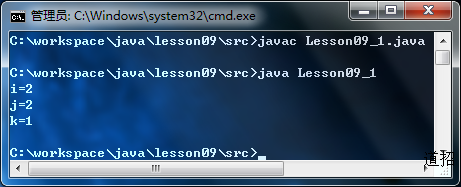我们可以看到，当方法内的局部和成员变量名字相同时，在方法内，局部变量遮蔽住了成员变量，因此打印出来的是2，而不是1。 再看一个子类成员变量遮蔽父类成员变量的例子。
 `01` `public` `class` `WhiteHorse ``extends` `Horse {`
 `02`
 `03` `    ``private` `static` `String color =``"白色"``;`
 `04`
 `05` `    ``public` `static` `int` `leg =``4``;`
 `06`
 `07` `    ``public` `static` `void` `main(String[] args){`
 `08`
 `09` `        ``WhiteHorse xiaobai = ``new` `WhiteHorse();`
 `10` `        ``System.out.println(xiaobai.color);`
 `11` `        ``System.out.println(xiaobai.leg);`
 `12`
 `13` `        ``//类变量是遮蔽不住的`
 `14` `        ``System.out.println(Horse.color);`
 `15`
 `16` `        ``//强制转换后我们看到父类的实体leg变量还在，只是被隐藏了`
 `17` `        ``Horse xiaobai1 = (Horse)xiaobai;`
 `18` `        ``System.out.println(xiaobai1.leg);`
 `19`
 `20` `    ``}`
 `21`
 `22` `}`

1. 参数列表必须与重写的方法的参数列表完全匹配（方法签名相同）。如果不匹配，你得到的将是方法重载。
2. 返回类型必须与超类中被重写方法中原先声明的返回类型或其子类型相同。
3. 访问级别的限制性可以比被重写方法弱，但是访问级别的限制性一定不能比被重写方法的更严格。
4. 仅当实例方法被子类继承时，它们才能被重写。子类和超类在同一个包内时，子类可以重写未标示为private和final的任何超类方法。不同包的子类只能重写标示为public或protected的非final方法。
5. 无论父类的方法是否抛出某种运行时异常，子类的重写方法都可以抛出任意类型的运行时异常。
6. 重写方法一定不能抛出比被重写方法声明的检验异常更新或更广的检验异常，可以抛出更少或更有限的异常。
7. 不能重写标示为final的方法。
8. 不能重写标示为static的方法。
9. 如果方法不能被继承，那么方法不能被重写。
我们举一个重写的例子： Horse.java
 `1` `public` `class` `Horse {`
 `2` `    ``//给马写个摆Pose的方法`
 `3` `    ``public` `void` `pose(){`
 `4` `        ``//样子很酷`
 `5` `        ``System.out.println(``"Cool!"``);`
 `6` `    ``}`
 `7` `}`
WhiteHorse.java
 `01` `public` `class` `WhiteHorse ``extends` `Horse {`
 `02`
 `03` `    ``//白马重写了摆pose的方法`
 `04` `    ``public` `void` `pose(){`
 `05` `        ``//白马更酷一点`
 `06` `        ``System.out.println(``"Cool!!!!"``);`
 `07` `    ``}`
 `08`
 `09` `    ``public` `static` `void` `main(String[] args){`
 `10` `        ``WhiteHorse xiaobai = ``new` `WhiteHorse();`
 `11` `        ``xiaobai.pose();`
 `12` `    ``}`
 `13` `}`

 `01` `public` `class` `Lesson09_1 {`
 `02`
 `03` `    ``int` `i=``1``;`
 `04` `    ``int` `j=``1``;`
 `05` `    ``int` `k=``1``;`
 `06` `    ``static` `int` `l = ``1``;`
 `07`
 `08` `    ``void` `test(``int` `i){`
 `09` `        ``int` `j=``2``;`
 `10` `        ``int` `l=``2``;`
 `11` `        ``System.out.println(``"i="``+i);`
 `12` `        ``System.out.println(``"j="``+j);`
 `13` `        ``System.out.println(``"k="``+k);`
 `14` `        ``System.out.println(``"l="``+l);`
 `15`
 `16` `        ``System.out.println(``"this.i="``+``this``.i);`
 `17` `        ``System.out.println(``"this.j="``+``this``.j);`
 `18` `        ``System.out.println(``"this.k="``+``this``.k);`
 `19` `        ``System.out.println(``"this.l="``+``this``.l);   `
 `20`
 `21` `    ``}`
 `22`
 `23` `    ``public` `static` `void` `main(String[] args){`
 `24` `        ``Lesson09_1 lesson = ``new` `Lesson09_1();`
 `25` `        ``lesson.test(``2``);`
 `26`
 `27` `    ``}`
 `28` `}`

 `01` `public` `class` `Lesson {`
 `02`
 `03` `    ``private` `int` `minute=``0``;`
 `04`
 `05` `    ``Lesson(){`
 `06` `        ``this``(``45``);`
 `07` `    ``}`
 `08`
 `09` `    ``Lesson(``int` `minute){`
 `10` `        ``this``.minute = minute;`
 `11` `    ``}`
 `12`
 `13` `    ``public` `static` `void` `main(String[] args){`
 `14` `        ``Lesson lesson = ``new` `Lesson();`
 `15` `        ``System.out.println(lesson.minute);`
 `16`
 `17` `        ``Lesson lesson2 = ``new` `Lesson(``30``);`
 `18` `        ``System.out.println(lesson2.minute);`
 `19` `    ``}`
 `20` `}`

 `01` `public` `class` `Horse {`
 `02`
 `03` `    ``public` `int` `height =``120``;`
 `04`
 `05` `    ``//给马写个摆Pose的方法`
 `06` `    ``public` `void` `pose(){`
 `07` `        ``//样子很酷`
 `08` `        ``System.out.println(``"Cool!"``);`
 `09` `    ``}`
 `10` `}`
WhiteHorse.java
 `01` `public` `class` `WhiteHorse ``extends` `Horse {`
 `02`
 `03` `    ``public` `int` `height =``150``;`
 `04`
 `05` `    ``//白马重写了摆pose的方法`
 `06` `    ``public` `void` `pose(){`
 `07`
 `08` `        ``//先摆一个马的pose`
 `09` `        ``super``.pose();`
 `10`
 `11` `        ``//白马更酷一点`
 `12` `        ``System.out.println(``"Cool!!!!"``);`
 `13` `    ``}   `
 `14`
 `15` `    ``public` `void` `printHeight(){`
 `16`
 `17` `        ``//打印父类被隐藏的变量`
 `18` `        ``System.out.println(``super``.height);`
 `19`
 `20` `        ``//打印实例变量`
 `21` `        ``System.out.println(height);`
 `22` `    ``}   `
 `23`
 `24` `    ``public` `static` `void` `main(String[] args){`
 `25` `        ``WhiteHorse xiaobai = ``new` `WhiteHorse();`
 `26` `        ``xiaobai.pose();`
 `27` `        ``xiaobai.printHeight();`
 `28`
 `29` `    ``}`
 `30` `}`

 `1` `public` `class` `Horse {`
 `2`
 `3` `    ``public` `Horse(){`
 `4` `        ``System.out.println(``"马类的构造函数"``);`
 `5` `    ``}`
 `6`
 `7` `}`
WhiteHorse.java
 `01` `public` `class` `WhiteHorse ``extends` `Horse {`
 `02`
 `03` `    ``public` `WhiteHorse(){`
 `04` `        ``System.out.println(``"白马类的构造函数"``);`
 `05`
 `06` `    ``}`
 `07`
 `08` `    ``public` `static` `void` `main(String[] args){`
 `09` `        ``new` `WhiteHorse();`
 `10` `    ``}`
 `11` `}`

 `01` `public` `class` `Horse {`
 `02`
 `03` `    ``protected` `int` `leg = ``0``;`
 `04`
 `05` `    ``public` `Horse(``int` `leg){`
 `06`
 `07` `        ``this``.leg=``4``;`
 `08`
 `09` `        ``System.out.println(``"马类的构造函数"``);`
 `10` `    ``}`
 `11`
 `12` `}`

 `01` `public` `class` `WhiteHorse ``extends` `Horse {`
 `02`
 `03` `    ``public` `WhiteHorse(){`
 `04` `        ``super``(``4``);`
 `05` `        ``System.out.println(``"白马类的构造函数"``);`
 `06` `    ``}`
 `07`
 `08` `    ``public` `static` `void` `main(String[] args){`
 `09` `        ``new` `WhiteHorse();`
 `10` `    ``}`
 `11` `}`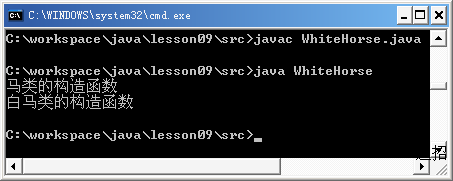### 相关文章

#### Java基础第十一讲：面向对象基础（五）

##### 关注道招网公众帐号##### 道招开发者二群